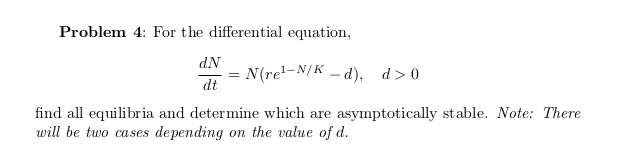# Problem 4: For the differential equation,dNN(rel-N/K – d),d>0%3Ddtfind all equilibria and determine which are asymptotically stable. Note: Therewill be two cases depending on the value of d.

Question
28 viewshelp_outlineImage TranscriptioncloseProblem 4: For the differential equation, dN N(rel-N/K – d), d>0 %3D dt find all equilibria and determine which are asymptotically stable. Note: There will be two cases depending on the value of d. fullscreen
check_circle

Step 1

The given differential equation is

Step 2

For the equilibria,

Step 3

For if N > 0 => dN/dt > 0 and if N < 0 =&...

### Want to see the full answer?

See Solution

#### Want to see this answer and more?

Solutions are written by subject experts who are available 24/7. Questions are typically answered within 1 hour.*

See Solution
*Response times may vary by subject and question.
Tagged in

### Math